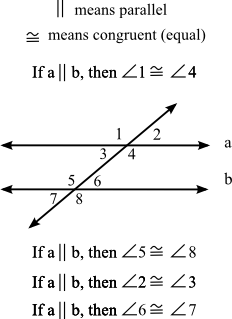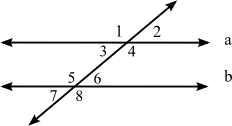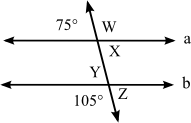# Parallel Lines and Transversals

Back to “Geometry” Page

Okay. Yes. This is stupid. But standardized tests like to know whether you know this. Don’t ask me why. Probably because it’s easy to make standardized tests with. Best guess. Anyway, you already know it. But you have probably forgotten it. Study the graphic below and then try the flashcards.## Now let’s mix it up.

• Angle 1 equals angle 2 (T/F)?
False
• Angle 1 equals angle 5 (T/F)?
True
• Angle 2 equals angle 4 (T/F)?
False
• Angle 2 equals angle 6 (T/F)?
True
• Angle 3 equals angle 4 (T/F)?
False
• Angle 3 equals angle 7 (T/F)?
True
• Angle 4 equals angle 5 (T/F)?
True
• Angle 4 equals angle 6 (T/F)?
False
• Angle 5 equals angle 6 (T/F)?
False
• Angle 5 equals angle 8 (T/F)?
True

Okay. Get ready. Remember that a straight line represents a 180° angle. And a pair of angles that meet on a straight line are “supplementary” and total 180°. Now try the next few flashcards.

Lines a and b are still parallel.• If angle 1 is 135°, then angle 2 is
45°
• If angle 3 is 45°, then angle 4 is
135°
• If angle 3 is 45°, then angle 7 is
45°
• If angle 1 is 135°, then angle 8 is
135°• The measure of angle W is
105°
• The measure of angle X is
75°
• The measure of angle Y is
75°
• The measure of angle Z is
75°
• The sum of angles W and X is
180°
• The sum of angles W and Z is
180°
• The sum of angles X and Y is
150°
• The sum of angles Y and Z is
150°